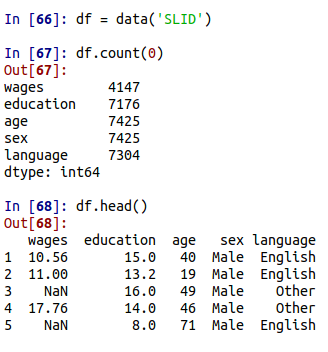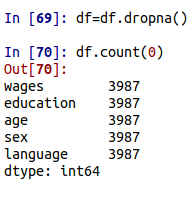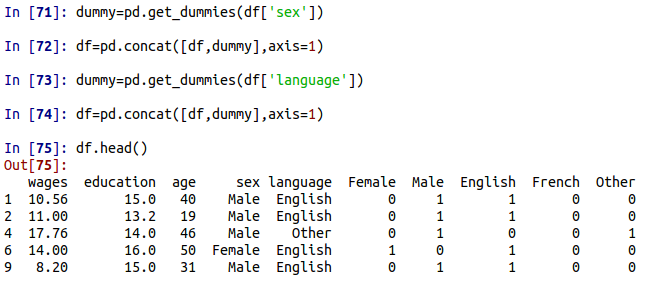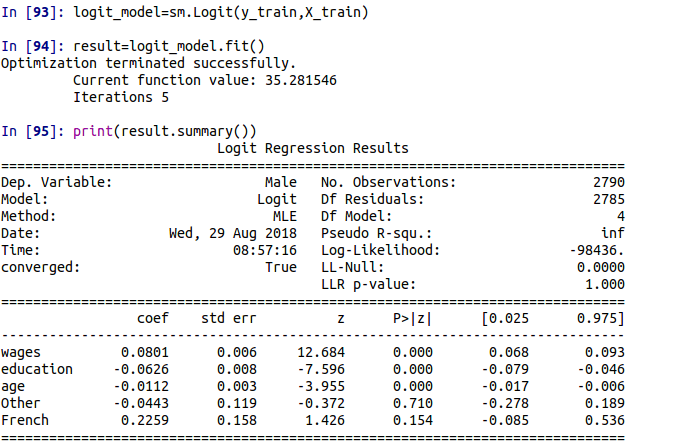Logistic Regression in Python

This post will provide an example of a logistic regression analysis in Python. Logistic regression is commonly used when the dependent variable is categorical.Our goal will be to predict the gender of an example based on the other variables in the model. Below are the steps we will take to achieve this.

1. Data preparation
2. Model development
3. Model testing
4. Model evaluation

Data Preparation

The dataset we will use is the ‘Survey of Labour and Income Dynamics’ (SLID) dataset available in the pydataset module in Python. This dataset contains basic data on labor and income along with some demographic information. The initial code that we need is below.

import pandas as pd
import statsmodels.api as sm
import numpy as np
import matplotlib.pyplot as plt
from sklearn.linear_model import LogisticRegression
from sklearn.model_selection import train_test_split
from sklearn import metrics
from pydataset import data

The code above loads all the modules and other tools we will need in this example. We now can load our data. In addition to loading the data, we will also look at the count and the characteristics of the variables. Below is the code.At the top of this code, we create the ‘df’ object which contains our data from the “SLID”. Next, we used the .count() function to determine if there was any missing data and to see what variables were available. It appears that we have five variables and a lot of missing data as each variable has different amounts of data. Lastly, we used the .head() function to see what each variable contained. It appears that wages, education, and age are continuous variables well sex and language are categorical. The categorical variables will need to be converted to dummy variables as well.

The next thing we need to do is drop all the rows that are missing data since it is hard to create a model when data is missing. Below is the code and the output for this process.In the code above, we used the .dropna() function to remove missing data. Then we used the .count() function to see how many rows remained. You can see that all the variables have the same number of rows which is important for model analysis. We will now make our dummy variables for sex and language in the code below.Here is what we did,

1. We used the .get_dummies function from pandas first on the sex variable. All this was stored in a new object called “dummy”
2. We then combined the dummy and df datasets using the .concat() function. The axis =1 argument is for combing by column.
3. We repeat steps 1 and 2 for the language variable
4. Lastly, we used the .head() function to see the results

With this, we are ready to move to model development.

Model Development

The first thing we need to do is put all of the independent variables in one dataframe and the dependent variable in its own dataframe. Below is the code for this

X=df[['wages','education','age',"French","Other"]]
y=df['Male']

Notice that we did not use every variable that was available. For the language variables, we only used “French” and “Other”. This is because when you make dummy variables you only need k-1 dummies created. Since the language variable had three categories we only need two dummy variables. Therefore, we excluded “English” because when “French” and “Other” are coded 0 it means that “English” is the characteristic of the example.

In addition, we only took “male” as our dependent variable because if “male” is set to 0 it means that example is female. We now need to create our train and test dataset. The code is below.

X_train, X_test, y_train, y_test = train_test_split(X, y, test_size=0.3, random_state=0)

We created four datasets

• train dataset with the independent variables
• train dataset with the dependent variable
• test dataset with the independent variables
• test dataset with the independent variable

The split is 70/30 with 70% being used for the training and 30% being used for testing. This is the purpose of the “test_size” argument. we used the train_test_split function to do this. We can now run our model and get the results. Below is the code.Here is what we did

1. We used the .Logit() function from statsmodel to create the logistic model. Notice we used only the training data.
2. We then use the .fit() function to get the results and stored this in the result object.
3. Lastly, we printed the results in the ‘result’ object using the .summary()

There are some problems with the results. The Pseudo R-square is infinity which is usually. Also, you may have some error output about hessian inversion in your output. For these reasons, we cannot trust the results but will continue for the sake of learning.

The coefficients are clear.  Only wage, education, and age are significant. In order to determine the probability you have to take the coefficient from the model and use the .exp() function from numpy. Below are the results.

np.exp(.08)
Out: 1.0832870676749586

np.exp(-0.06)
Out: 0.9417645335842487

np.exp(-.01)
Out: 0.9900498337491681

For the first value, for every unit wages increaser the probability that they are male increase 8%. For every 1 unit increase in education there probability of the person being male decrease 6%. Lastly, for every one unit increase in age the probability of the person being male decrease by 1%. Notice that we subtract 1 from the outputs to find the actual probability.

We will now move to model testing

Model Testing

To do this we first test our model with the code below

y_pred=result.predict(X_test)

We made the result object earlier. Now we just use the .predict() function with the X_test data. Next, we need to flag examples that the model believes has a 60% chance or greater of being male. The code is below

y_pred_flag=y_pred>.6

This creates a boolean object with True and False as the output. Now we will make our confusion matrix as well as other metrics for classification.The results speak for themselves. There are a lot of false positives if you look at the confusion matrix. In addition precision, recall, and f1 are all low. Hopefully, the coding should be clear the main point is to be sure to use the test set dependent dataset (y_test) with the flag data you made in the previous step.

We will not make the ROC curve. For a strong model, it should have a strong elbow shape while with a weak model it will be a diagonal straight line.The first plot is of our data. The second plot is what a really bad model would look like. As you can see there is littte difference between the two. Again this is because of all the false positives we have in the model. The actual coding should be clear. fpr is the false positive rate, tpr is the true positive rate. The function is .roc_curve. Inside goes the predict vs actual test data.

Conclusion

This post provided a demonstration of the use of logistic regression in Python. It is necessary to follow the steps above but keep in mind that this was a demonstration and the results are dubious.